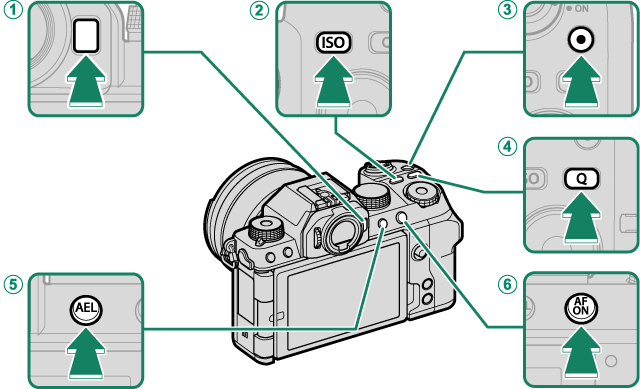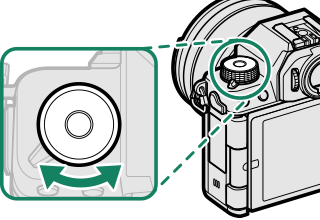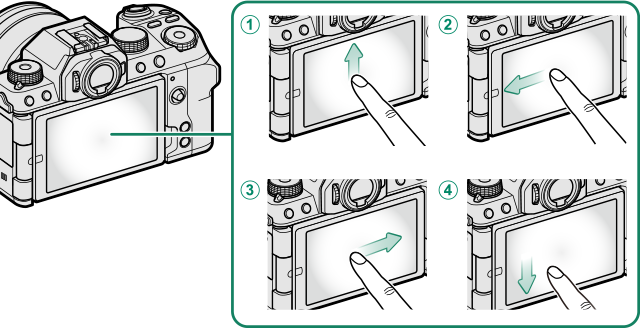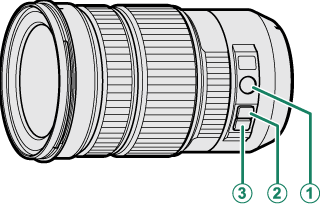## 功能按钮

### 功能按钮默认设定AFn 按钮

BISO 按钮

ISO

Ct（动画录制）按钮

DQ（快速菜单）按钮

EAEL（曝光锁定）按钮

FAFON 按钮

AF-ON

### 为功能按钮指定功能

• 图像尺寸
• 图像质量
• RAW
• 胶片模拟
• 颗粒效果
• 色彩效果
• 彩色FX蓝色
• 动态范围
• D范围优先级
• 白平衡
• 清晰度
• 聚焦区域
• 对焦确认
• 对焦模式
• 自动对焦模式
• AF-C 自定设置
• 脸部识别开/关
• 对焦范围限制器
• 焦点检查锁定
• 运动取景器模式
• 预拍摄 sJ
• 自拍
• AE连拍设置
• 对焦包围设定
• 测光
• 快门类型
• 减少闪烁
• ISO
• 防抖模式
• 无线通信
• 闪光补偿
• 闪光灯功能设置
• TTL-锁定
• 模型化闪光灯
• 视频录制释放
• 全HD高速录制
• 视频裁剪放大 C
• F 图像稳定模式增强
• 斑纹设置
• 内置/外置麦克风音量调节
• 4通道音频播放
• 视频优化控制 oL
• VIEW MODE设置
• 景深预览
• 手动模式下预览曝光/白平衡
• 自然实时视图
• 直方图
• 电子水平仪
• 大尺寸指示器模式
• F-Log查看助手
• 变焦/对焦控制环
• 匀速变焦(T)
• 匀速变焦(W)
• F恒速对焦(N)
• F匀速对焦(F)
• 仅限 AE 锁
• 仅限 AF 锁
• AE/AF 锁
• AF-ON
• 仅AWB锁定
• 锁定设定
• 性能
• 自动图像传输
• 选择配对目的地
• Bluetooth 开/关
• 快捷菜单
• 播放

## 功能拨盘• 曝光补偿
• 图像尺寸
• 图像质量
• 胶片模拟
• 颗粒效果
• 色彩效果
• 彩色FX蓝色
• 动态范围
• D范围优先级
• 白平衡
• 清晰度
• 对焦模式
• 自动对焦模式
• AF-C 自定设置
• 脸部识别开/关
• 对焦范围限制器
• 运动取景器模式
• 预拍摄 sJ
• 自拍
• 测光
• 快门类型
• 减少闪烁
• ISO
• 防抖模式
• 闪光补偿
• 闪光灯功能设置
• 全HD高速录制
• 视频裁剪放大 C
• F 图像稳定模式增强
• 斑纹设置
• 内置/外置麦克风音量调节
• 视频优化控制 oL
• 默认
• 默认值(d，设置为B时)

## 触摸功能动作

### 触控功能动作默认设置AT-Fn1（向上轻拨）

BT-Fn2（向左轻拨）

CT-Fn3（向右轻拨）

DT-Fn4（向下轻拨）

### 为触控功能动作指定功能

• 图像尺寸
• 图像质量
• RAW
• 胶片模拟
• 颗粒效果
• 色彩效果
• 彩色FX蓝色
• 动态范围
• D范围优先级
• 白平衡
• 清晰度
• 聚焦区域
• 对焦确认
• 对焦模式
• 自动对焦模式
• AF-C 自定设置
• 脸部识别开/关
• 对焦范围限制器
• 焦点检查锁定
• 运动取景器模式
• 预拍摄 sJ
• 自拍
• AE连拍设置
• 对焦包围设定
• 测光
• 快门类型
• 减少闪烁
• ISO
• 防抖模式
• 无线通信
• 闪光补偿
• 闪光灯功能设置
• TTL-锁定
• 模型化闪光灯
• 全HD高速录制
• 视频裁剪放大 C
• F 图像稳定模式增强
• 斑纹设置
• 内置/外置麦克风音量调节
• 4通道音频播放
• 视频优化控制 oL
• VIEW MODE设置
• 景深预览
• 手动模式下预览曝光/白平衡
• 自然实时视图
• 直方图
• 电子水平仪
• 大尺寸指示器模式
• F-Log查看助手
• 变焦/对焦控制环
• 锁定设定
• 性能
• 自动图像传输
• 选择配对目的地
• Bluetooth 开/关
• 快捷菜单
• 播放

## 镜头功能按钮

### 镜头功能按钮默认设定AZ/F

BL-Fn1（向上按拨动变焦按钮）

CL-Fn2（向下按拨动变焦按钮）

### 为镜头功能按钮指定功能

• 图像尺寸
• 图像质量
• RAW
• 胶片模拟
• 颗粒效果
• 色彩效果
• 彩色FX蓝色
• 动态范围
• D范围优先级
• 白平衡
• 清晰度
• 聚焦区域
• 对焦确认
• 对焦模式
• 自动对焦模式
• AF-C 自定设置
• 脸部识别开/关
• 对焦范围限制器
• 焦点检查锁定
• 运动取景器模式
• 预拍摄 sJ
• 自拍
• AE连拍设置
• 对焦包围设定
• 测光
• 快门类型
• 减少闪烁
• ISO
• 防抖模式
• 无线通信
• 闪光补偿
• 闪光灯功能设置
• TTL-锁定
• 模型化闪光灯
• 视频录制释放
• 全HD高速录制
• 视频裁剪放大 C
• F 图像稳定模式增强
• 斑纹设置
• 内置/外置麦克风音量调节
• 4通道音频播放
• 视频优化控制 oL
• VIEW MODE设置
• 景深预览
• 手动模式下预览曝光/白平衡
• 自然实时视图
• 直方图
• 电子水平仪
• 大尺寸指示器模式
• F-Log查看助手
• 变焦/对焦控制环
• 匀速变焦(T)
• 匀速变焦(W)
• F恒速对焦(N)
• F匀速对焦(F)
• 仅限 AE 锁
• 仅限 AF 锁
• AE/AF 锁
• AF-ON
• 仅AWB锁定
• 锁定设定
• 性能
• 自动图像传输
• 选择配对目的地
• Bluetooth 开/关
• 快捷菜单
• 播放